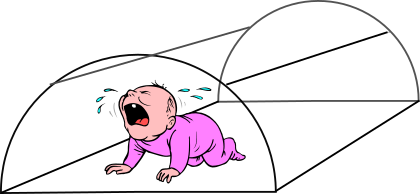# Exam-Style Questions on Optimisation

## Problems on Optimisation adapted from questions set in previous Mathematics exams.

### 1.

IB Studies

A child's play tent is made in the shape of half a cylinder. It is constructed from a fibreglass frame with material pulled tightly around it. The fibreglass frame consists of a rectangular base, two semi-circular ends and two further support rods, as shown in the following diagram.The semicircular ends each have radius $$r$$ and the support rods each have length $$d$$.

Let F be the total length of fibreglass used in the frame of the play tent.

(a) Write down an expression for $$F$$ in terms of $$r$$, $$d$$ and $$\pi$$.

The volume of the play tent is 0.95 m3.

(b) Write down an equation for the volume of the play tent in terms of $$r$$, $$d$$ and $$\pi$$.

(c) Show that $$F = 2\pi r + 4r + \frac{7.6}{\pi r^2}$$

(d) Find $$\frac{dF}{dr}$$

The play tent is designed so that the length of fibreglass used in its frame is a minimum.

(e) Find the value of $$r$$ for which $$F$$ is a minimum.

(f) Calculate the value of $$d$$ for which $$F$$ is a minimum.

(g) Calculate the minimum value of $$F$$.

### 2.

IB Studies

A package is in the shape of a cuboid and has a length $$l$$ cm, width $$w$$ cm and height of 12 cm.

(a) Express the volume of the package in terms of $$l$$ and $$w$$.

The total volume of the package is 2400 cm3.

(b) Show that $$l=\frac{200}{w}$$.

The package is tied up using a length of red string that fits exactly around the package in two different directions, as shown in the following diagram (not to scale).(c) Show that the length of string, $$x$$cm, required to tie up the package can be written as $$24+4w+\frac{400}{w}$$

(d) Sketch the graph of $$x$$ for $$0\lt w \le 12$$, clearly showing the local minimum point.

(e) Find $$\frac{dx}{dw}$$.

(f) Find the value of $$w$$ for which $$x$$ is a minimum.

(g) Find the value, $$l$$, of the package for which the length of string is a minimum.

(h) Find the minimum length of string required to tie up the package.

### 3.

A-Level

Moresum Soup is sold in cans with a capacities of 400ml each. Each can is in the shape of a right circular cylinder with radius $$r$$ cm and height $$h$$ cm.

(a) Assuming that the can is made from a metal of negligible thickness prove that the total surface area, A cm2, of the can is given by the following formula:

$$A= 2 \pi r^2 + \frac{800}{r}$$

(b) Given that r can vary, find the dimensions of a can that has minimum surface area.

If you would like space on the right of the question to write out the solution try this Thinning Feature. It will collapse the text into the left half of your screen but large diagrams will remain unchanged.

The exam-style questions appearing on this site are based on those set in previous examinations (or sample assessment papers for future examinations) by the major examination boards. The wording, diagrams and figures used in these questions have been changed from the originals so that students can have fresh, relevant problem solving practice even if they have previously worked through the related exam paper.

The solutions to the questions on this website are only available to those who have a Transum Subscription.

Exam-Style Questions Main Page

Search for exam-style questions containing a particular word or phrase:

To search the entire Transum website use the search box in the grey area below.